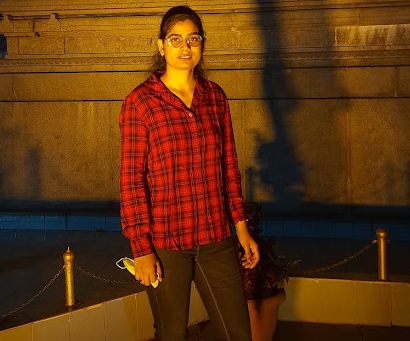Kajal Kumari — February 24, 2023Published On

## Introduction

An image is a two-dimensional representation of a visual subject, like a photograph, painting, or drawing. In digital imaging, images are stored as arrays of pixel values, where each pixel represents a sample of the image’s brightness and color. The color of each pixel can be represented by one or multiple channels, like the red, green, and blue (RGB) channels in traditional color images. In this article, you will learn various image preprocessing techniques.

Images can be processed using computer algorithms to change their appearance or extract information. Image processing techniques include operations like resizing, cropping, rotating, filtering, and thresholding. These operations are performed on the pixel values to modify the image or extract information about its content. Image processing is used in so many applications, including computer vision, medical imaging, and digital art.

By learning Image Preprocessing using SKimage, you will be able to:

1. Understand the importance of image preprocessing in image analysis and machine learning.
2. Learn how to use various SKimage functions for image filtering, enhancement, restoration, and transformation.
3. Apply image preprocessing techniques such as noise reduction, edge detection, and image thresholding to improve image quality.
4. Perform common image preprocessing tasks such as image resizing, cropping, and rotation.
5. Use feature extraction techniques to extract meaningful information from images.
6. Implement object detection algorithms for image analysis applications.

This article was published as a part of the Data Science Blogathon.

## Understanding the Image data

Let’s go with an image that can be broken down into a matrix of numbers, where each number represents the strength. And this strength could be anywhere between 0 (representing black) and 255 (representing white). So, a monochromatic image can be represented by a single matrix.

But what can we do when it is a colored image below?If we have to represent an image, we will break it into three images of three different colors: red, green, and blue. We can store the intensities of each color in two single matrices.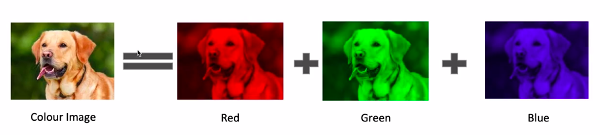So the image will be broken down into three matrices: one for the red color, one for the green color, and one for the blue color so that we can represent the image in an N*M*3 matrix.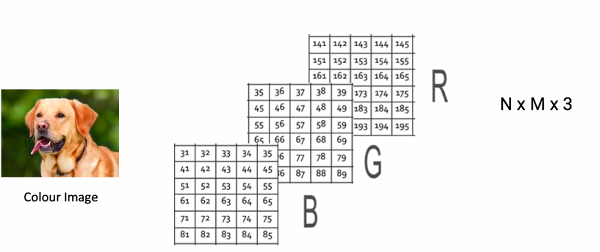Any image that is n*m pixels wide can always be defined as a matrix N*M*3 anywhere in the computer.

When processing image data, it is common to convert the image into a numerical representation, like a matrix, so that computer algorithms can process it. The numerical representation of the image is called a digital image, and the data in the digital image can be manipulated using mathematical operations to perform different image-processing tasks.

Overall, understanding image data is necessary for working with image processing algorithms and extracting information from images.

## Steps Involved in Processing an Image

Here are the common steps involved in processing an image in Python:

1. Importing libraries: You need to import the libraries that you will use to process the image, like NumPy and OpenCV.
3. Pre-processing: Depending on the image and the desired outcome, you may need to perform pre-processing steps like resizing, grayscaling, or thresholding.
4. Manipulating pixels: You can manipulate the pixels of the image using NumPy arrays to perform operations like cropping, rotating, and making color-based selections.
5. Filtering: You can use different filters to smooth or sharpen the image, like Gaussian or median filters.
6. Edge detection: Edge detection, which can be performed using methods such as Canny, is used to identify boundaries between objects in an image.

## Understanding Transformations in Images

Transformations in images refer to mathematical operations applied to an image to change its appearance or extract useful information from it. Many types of transformations can be applied to images, including:

1. Geometric transformations: These transformations change the spatial relationship between pixels in an image, like rotation, scaling, and translation.
2. Color transformations: These transformations change the color properties of an image, like brightness, contrast, and saturation.
3. Filtering: Filtering refers to the process of removing noise from an image or enhancing its features, like smoothing or sharpening.
4. Edge detection: Edge detection, which can be performed using methods such as Canny, is used to identify boundaries between objects in an image.
5. Feature extraction: This refers to the process of extracting meaningful information from an image, like corners or key points, using algorithms like Harris corner detection or SIFT.

These transformations are performed using mathematical algorithms and can be implemented in software like Python or MATLAB. Transformations are essential to image processing and are crucial in applications like computer vision, medical image analysis, and facial recognition.

Now we will start to load the image and do some operations on the image using the Scikit Image Library.

## How to Load an Image?

Depending on the programming language and tools being used, there are several ways to load an image. Here are a few common ways:

1. Using an image processing library: Many image processing libraries, like OpenCV, Pillow, and Scikit-Image, provide functions to load images into memory. For example, you can use the imread function to load an image in OpenCV.
2. Using an image file reader: You can use a function or class specific to a file format (like JPEG or PNG) to read the image data from a file. For example, you can use the imageio library in Python to read image files.

Once the image is uploaded into memory, you can perform various operations on it, like resizing, cropping, color conversion, and filtering, using the functions provided by the image processing library.

Here is an example of how to load an image using the scikit-image (skimage) library in Python:

``````originating from Skimage Import IO
# Open the image
# Show the image
io.imshow(image)
io.show()``````

In this example, the imread function from the io module of the skimage library is used to load an image file called image.jpg into memory. The resulting image data is then displayed using the imshow function from the io module, followed by a call to “io.show()” to display the image. The imshow function automatically adjusts the image for display and handles issues like color channels and aspect ratio.

## How to Visualize an Image?

Visualizing an image involves displaying the image data on a screen or output device. Visualizing an image depends on the programming language and tools being used. Here are a few common ways:

1. Using an image processing library: Many image processing libraries, like OpenCV, Pillow, and scikit-image, provide functions to display images. For example, you can use the imshow function to display an image in OpenCV.
2. Using a plotting library: You can use a plotting library, like Matplotlib in Python, to display an image. For example, you can use the imshow function from the Matplotlib Pyplot module to display an image in Python.

Once the image is displayed, you can interact with it by zooming in and out, panning, and readjusting the display settings.

Here is an example of how to visualize an image using the scikit-image (skimage) library in Python:

``````from skimage import io
import matplotlib.pyplot as  plt
# Display the image
plt.imshow(image)
plt.show()``````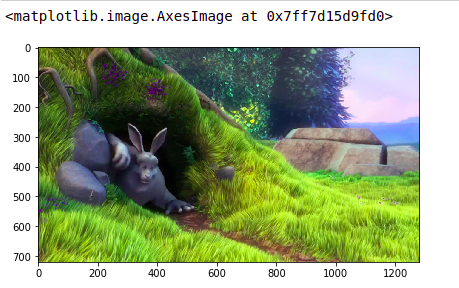In this example, the `imread` function from the `"io` ” module of the skimage library is used to load an image file called `image.jpg` into memory. The resulting image data is then displayed using the `imshow` function from the `matplotlib.pyplot` module, followed by a call to `plt.show()` to display the image.

## Image Preprocessing – Resizing Images

Python’s “scikit-image” (skimage) library provides several functions for resizing images. One commonly used function for this purpose is “resize” from the “transform” module.

Here is an example of using skimage to resize an image:

import skimage

from skimage import io,  transform

resized_image = transform.resize(image, (300, 300))

# Save the resized image
io.imsave(“resized_image.jpg”, resized_image)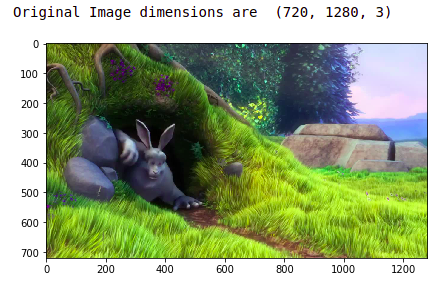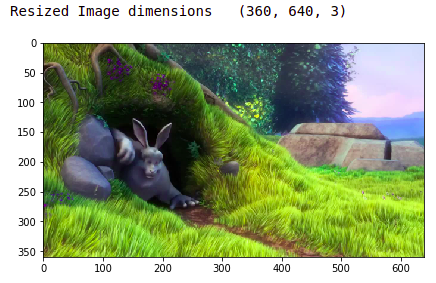In this example, the original image is read using the `imread` function from the `io` module. The `resize` the function is then used to resize the image to a size of (300 300) pixels. Finally, the resized image is saved using the `imsave` function.

## Image Preprocessing – Reshaping an Image

In Python, the “scikit-image” (skimage) library provides several functions for reshaping images. Here is an example of using skimage to reshape an image:

``````# import colour sub-module
from skimage import color``````
``````# reading the image
``````# converting image to grayscale
grayscale_image = color.rgb2gray(image)
grayscale_image.shape``````
``````import numpy as np
new_shape = (grayscale_image.shape*grayscale_image.shape)``````
``````# reshape
image2 = np.reshape(grayscale_image, new_shape)
image2.shape``````

If converting a 4 by 4 2-D image to 1-D, we will have 4×4=16 values.

## Image Preprocessing – Image Rotation

Image rotation involves rotating an image about its center by a specified angle. In scikit-image, you can use the `rotate` function from the `transform` module to rotate an image. Here’s an example in Python:

``````import numpy as np
from skimage import io
from skimage.transform import rotate``````
``````# Load an image
``````# Rotate the image by 180 degrees
rotated_image = rotate(image, angle=180, resize=True)``````
``````# Save the rotated image
io.imsave("rotated_image.jpg", rotated_image)``````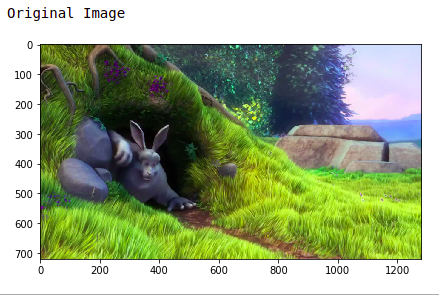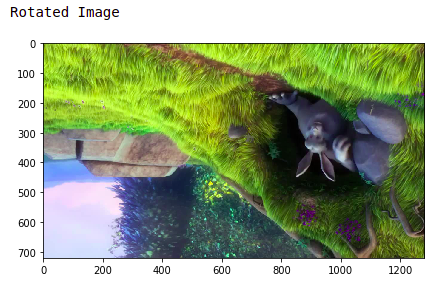In this example, the `rotate` function is used to rotate the input image by 180 degrees. The first argument to the `rotate` function is the input image, and the `angle` argument specifies the rotation angle in degrees.

## Image Preprocessing – Image Cropping

Image cropping involves extracting a portion of an image by specifying a crop region. In scikit-image, you can use slicing and indexing to crop an image. Here’s an example in Python:

``````import numpy as np
from skimage import io``````
``````# Load an image
rows, cols = image.shape[:2]
cropped_image = image[rows//4:-rows//4, cols//4:-cols//4]``````
``````# Save the cropped image
io.imsave("cropped_image.jpg", cropped_image)``````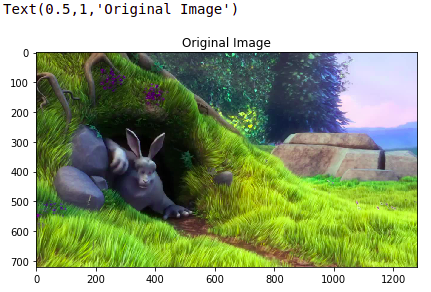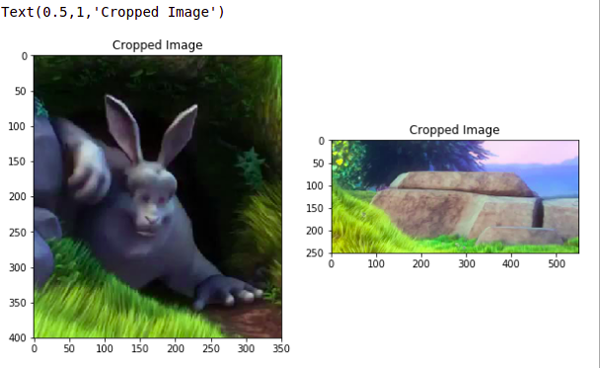In this example, the input image is first loaded using the `imread `function. The crop region is specified by slicing the image along both dimensions, such that the first and last quarter of the rows and columns are removed.

## Image Preprocessing – Image Flipping

Image flipping in Python can be performed using the “cv2.flip” function from the OpenCV library. The “cv2.flip” function takes two arguments: the input image and a flip code. The flip code specifies the flipping to be performed and can be one of the following values:

• cv2.FLIP_HORIZONTAL: Flip the image horizontally
cv2.FLIP_VERTICAL: Flip the image vertically
cv2.FLIP_BOTH: Flip the image both horizontally and vertically

Flipping can be considered an extension of rotation, allowing left-right and up-down image flipping.

``````import numpy as np
import matplotlib.pyplot as plt
%matplotlib inline``````
``````# reading the image
image = np.array(image)``````
``````imshow(image)
plt.title('Original Image')``````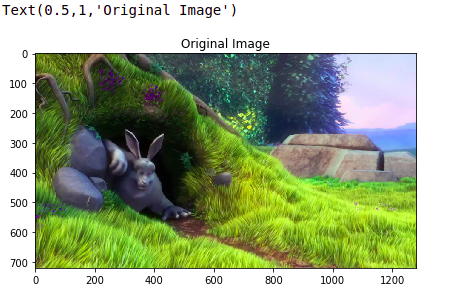Now, what do you do if you have to flip the images, again read the image and now in order to flip it? let’s say we are doing left to right flip. I can easily do that using the “fliplr()” function.

``````# flip image left-to-right
flipLR = np.fliplr(image)``````
``````plt.imshow(flipLR)
plt.title('Left to Right Flipped')``````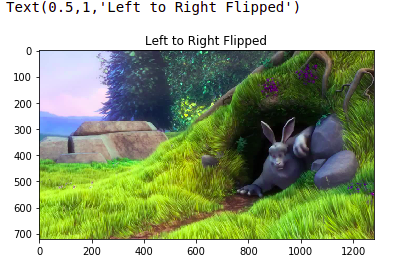And these are the ways in which you can flip the images.

## Image Preprocessing – Brightness Manipulation

Brightness manipulation in Python can be performed using the image library. The image library provides the exposure module, which includes the function of adjusting gamma that can be used to change the brightness of an image.

Images with different brightness can be used to make the model robust to changes in lighting conditions; this is important for systems that work in outdoor lightings, like CCTV cameras on traffic signals.

``from skimage.exposure import adjust_gamma``
``````# read the image
``````plt.title('Original Image')
imshow(image)``````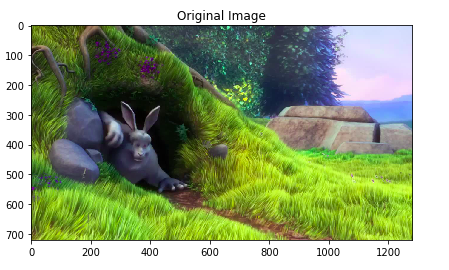I am going to change the gamma value, and that changes the strength of the image. So, this is my bright image.

``````# brighten the image
``````imshow(bright)
plt.title('Brightened IMage')``````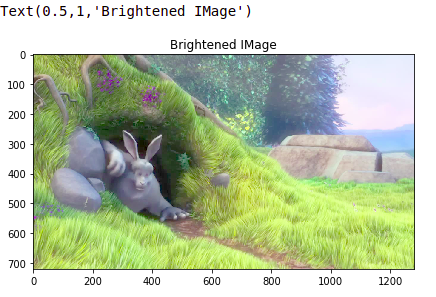So, approximately I can make all of these changes to the image.

## Conclusion

Scikit-image is a popular Python library for image processing that provides tools and functions for working with images. Here’s a summary of some of the critical features of image for image processing:

1. Image I/O: image provides functions for reading and writing images to disk, including `imread` for reading an image and `imsave` for saving an image.
2. Image restoration: image provides algorithms for restoring degraded images, including functions for removing noise and correcting for blurring or distortion.
3. Image analysis: image provides functions for analyzing image properties, including histograms, gradient magnitude, and texture analysis.
4. Image visualization: image provides functions for visualizing images and their properties, including plotting and displaying images, histograms, and other visual representations of image data.

Overall, image is a comprehensive and well-documented library for image processing that is widely used in scientific, medical, and industrial applications.